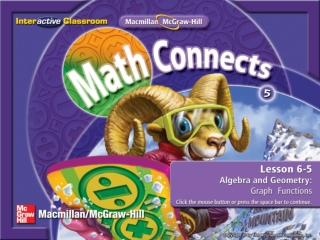DownloadDownload PresentationSplash Screen

# Splash Screen

Télécharger la présentation## Splash Screen

- - - - - - - - - - - - - - - - - - - - - - - - - - - E N D - - - - - - - - - - - - - - - - - - - - - - - - - - -
##### Presentation Transcript

1. Splash Screen

2. Five-Minute Check (over Lesson 6–4) Main Idea and Vocabulary Example 1: Graphing Ordered Pairs Example 2: Graphing Ordered Pairs Example 3: Graphing Functions Lesson Menu

3. I will graph points on a coordinate grid. • graph Main Idea/Vocabulary

4. Graphing Ordered Pairs Graph and label point G(5, 2) on the coordinate grid. Step 1 Start at the origin (0, 0). Step 2 Move 5 units to the right on the x-axis. G Step 3 Then move up 2 units to locate the point. Step 4 Draw a dot and label the point G. Example 1

5. A B C D A. B. C. D. Which shows point Q(1, 3) graphed on the coordinate grid? Example 1

6. Graphing Ordered Pairs Graph and label point C(3, 5) on the coordinate grid. Step 1 Start at the origin (0, 0). C Step 2 Move 3 units to the right on the x-axis. Step 3 Then move up 5 units to locate the point. Step 4 Draw a dot and label the point C. Example 2

7. A B C D A. B. C. D. Which shows point Q(4, 2) graphed on the coordinate grid? Example 2

8. Graphing Functions Every package of pencils Li bought cost \$2. Given the function rule 2p, find the total cost of 1, 2, 3, and 4 packages of pencils. Make a function table and then graph the ordered pairs. The function rule is 2p. Multiply the number of packages by 2 to find the total cost. Example 3

9. Graphing Functions Now graph the ordered pairs. (4, 8) (3, 6) (2, 4) (1, 2) Example 3

10. Every box of crayons Ying bought cost \$1. Given the function rule 3x, find the total cost of 2, 3, and 4 boxes of crayons. Which graph shows the corresponding ordered pairs? Example 3

11. A B C D A. B. C. D. Example 3

12. End of the Lesson End Lesson

13. Five-Minute Check (over Lesson 6–4) Image Bank Math Tool Chest Model Multiplication Equations Resources

14. A B C D (over Lesson 6–4) Name the ordered pair for point A. • (3, 3) • (2, 0) • (5, 1) • (1, 1) Five Minute Check 1

15. A B C D (over Lesson 6–4) Name the ordered pair for point D. • (1, 5) • (2, 0) • (3, 3) • (5, 1) Five Minute Check 2

16. A B C D (over Lesson 6–4) Name the point for (0, 2). A.A B. B C. C D. D Five Minute Check 3

17. A B C D (over Lesson 6–4) Name the point for (1, 5). A.A B. B C. C D. D Five Minute Check 4

18. End of Custom Shows